## Introduction

Convolutional neural networks (also called CNNs or ConvNets) are neural networks used traditionally to classify images, for example to identify faces, objects, street signs, tumors,… They have the ability to recognise any kind of data if they get trained with a suitable dataset.

CNNs are not limited to image recognition, they have been applied directly to Natural Language Processing, sounds  to Automated Speech Recognition and to various numerical datasets.

Neural networks take their metaphor from the brain and its neurons. The brain contains more than 80 billions of neurons and approximately connections or synapses. As shown in the bellow drawing, neurons are complex and all their complexity cannot be captured by a simple mathematical model;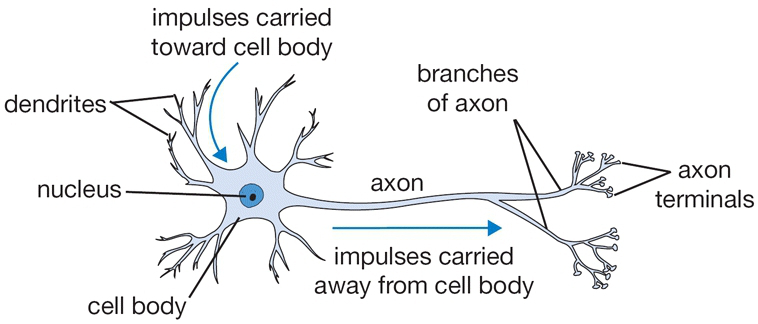Yet most of the striking abilities of the brain can be captured by representing the neuron as a simple aggregating and thresholding unit. Basic biology courses tell us that the neuron main function (and not the only function) is is to respond to the incoming excitatory and inhibitory stimulation, aggregate them up and fire back if this aggregation exceeds a certain threshold. A simple and effective representation of this phenomena is showed in the bellow representation:The neurone computes the weighted sum of its inputs and fires if this sum is above a certain threshold. Its firing rate depends on the strength of the inputs. The firing rate is computed by an activation function. This activation function is important because it gives the system its non linear behaviour.

There are several types activation functions. The following table summarises the most important ones:

 Function Graph Description Sigmoid or Logistic Activation Function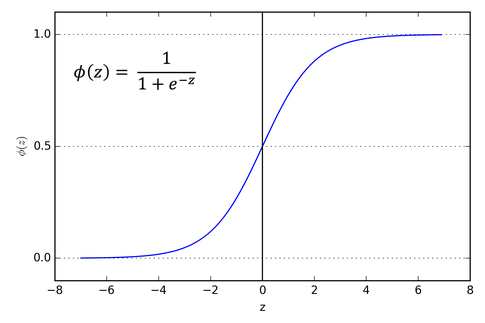The Sigmoid Function curve looks like a S-shape. Advantages: maps nicely with probabilities as it varies from 0 to 1. Tanh or hyperbolic tangent Activation Function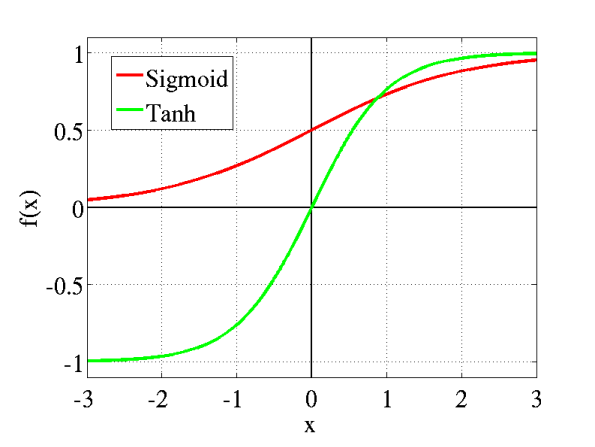Tanh is also like logistic sigmoid but better. The range of the tanh function is from (-1 to 1). tanh is also sigmoidal (s – shaped). Advantages: In this function the negative inputs are mapped strongly to negative values and the zero inputs will be mapped near zero in the tanh graph. ReLU (Rectified Linear Unit) Activation Function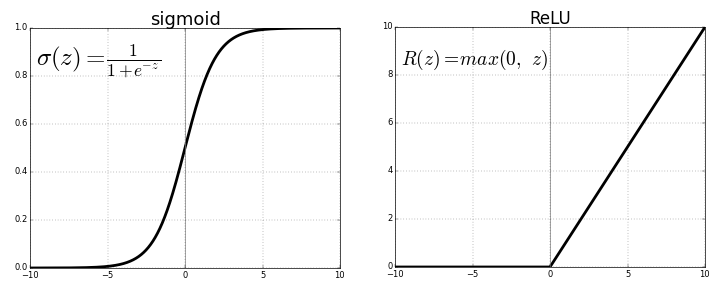This is the most used activation function in the world right now.Since, it is used in almost all the convolutional neural networks or deep learning. Disadvantage: The negative values become zero immediately which decreases the ability of the model to fit or train from the data properly Leaky ReLU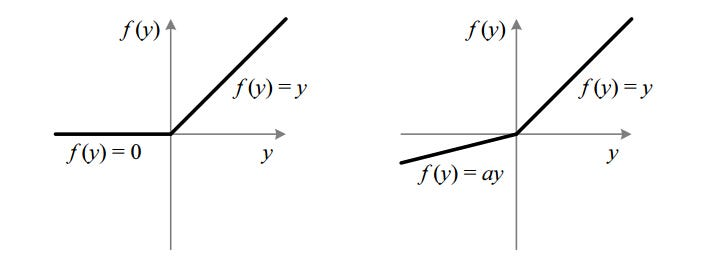It is an attempt to solve the dying ReLU problem. The leak helps to increase the range of the ReLU function. Usually, the value of a is 0.01 or so.

## Overview of Convolutional Neural Networks

The core building block of neural networks is the layer. The layer process data like a filter. Data goes in, and it comes out in another form. Layers extract representations out of the data and feed it to the next layer. Most of deep learning consists of chaining together such layers.

More specifically, the architecture of the CNN is shown in the following figure.

##• The Input layer: contain the input data – sound, text or image
• Convolution layer: perform  a convolution operation on the input (hence the name of the architecture)
• Pooling layer: Reduces the size of the input via a pooling operation, typically average or max.
• Fully connected layer: serves as a classifier.
• Softmax: Logistic is used for binary classification and softmax is for multi-classification.
• Output layer: Represents the classes.

Every layer has a specific function and feed into the next layer.

The convolution operation changes the representation of an image, starting from raw pixels at one end, to a higher level representation using successive convolution layers. As the system goes through multiple Convolutional Layers, it is basically performing non-linear transformations so similar  images are clustered closer to each other. This enables the classification operation to happen using a simple linear classifier in the final layer of the network. The Fully Connected layer that is just prior to the Output Layer, is called the Fully Connected layer, and it contains a high-dimensional representation of the input image. Adding Fully Connected layers to a ConvNet leads to an enormous increase in the number of parameters.

## Fundamental concepts

### Input layer:From Scalars to TensorsCNNs  ingest and process data as tensors, and tensors are matrices of numbers of higher dimensions.

A scalar is just a number, such as 1. scalars have 0 dimensions

A vector is a list of numbers (e.g., [1,2,3,4]). Vectors have 1 dimension

A matrix is a rectangular grid of numbers with rows and columns. A matrix is a two-dimensional plane,

A Tensor is a stacking of 2D matrices and it is a three-dimensional structure. Technically, they are arrays within arrays. Because these arrays can be nested inside each other, the number of dimension can be more than 3 and can grow indefinitely.

Tensors are a suitable representation of data structures for deep learning. An image for example can be thought of as 3 dimensional tensor with Width, height (the spatial dimensions) and depth (RGB colors) values.This structure can be mapped in several domains. In sound the dimensions can represent Audio Channels, Sample, rate and Bit-depth,  in text the dimensions can be words (see Word2vec) and the channels can represent various embedding types (Fasttext, glove, ..) or other analyses such as entities and Part Of Speech.

### Convolution Layer

The Convolution layer is where the convolution happens. It contains several new and potentially confusing concepts that we will explain first.

#### Understanding Convolution

Mathematically, a convolution is a mathematical operation on two functions (f and g) that produces a third function  h, expressing how the shape of one is modified by the other.

Convolution refers to both the result function and to the process of applying it.

ACredit: Mathworld.

The green curve is the result of the convolution of the blue and red curves as a function of t, it also shows how the convolution process is applied over all values of t. it may seem that this is a time based process, but it is not. The animations shows time but you can also consider t as projection over one of the space dimension. The result is the static green curve. It represents the integral of the area under the grey curve.  So in a sense, the two functions are being “rolled together.”

Below, is another visualisation the convolution of two box functions: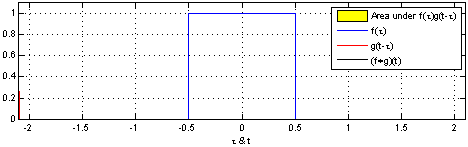From Wikipedia

Armed with this perspective, a lot of things become more intuitive.

If we keep the image analysis in mind (after all the concept mostly apply there), the static curve, is the image being analysed, and the second, mobile function is known as the filter, because it picks up a signal or feature in the image. As you can see from the animations, the convolution process is capturing specific features, such as the existence and the form of some shapes.

The “Stride” is the main parameter that controls the convolution. It define the way the filter is moved across the input. The filter is applied along all the dimensions of the input and we can choose how we apply it. For example if the filter is a magnifying loop and the image is a journal then you can choose to move the filter (the magnifying loop) slowly focussing on a word by word basis or jump form section to section if the loop field is large. The following figures show a filter moving with stride of “1” and a stride of “2”.With a stride of 1 we are moving the filter pixel per pixel and consequently with a stride of 2 we are moving the filter 2 pixels at each step.

In the above figure the filter is positioned at the top left corner and it will be moved until the top right corner of the filter will coincide with top right corner of the image. This means that the filter my miss a feature if this feature happens to to be in the first pixel. Think about our magnifying loop only magnifying at the center of the loop and we are not able to see the start of the word if we do not move the loop further to the left. This is where the padding is needed.

Padding is adding empty (or random) pixels at the edges in such a way that the filter can pass over the corner pixels several times during the convolution process.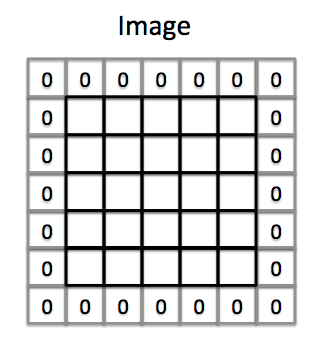### How the Convolution Layer works:

For each pixel of an image, the intensity of R, G and B will be expressed by a number (X11, X12,… in the following graph), and that number will be an element in one of the three, stacked two-dimensional matrices, which together form the image volume.The ConvNets purpose is to find which of those numbers are significant signals that actually help it classify images more accurately.

Rather than focus on one pixel at a time, a convolutional net takes in square patches of pixels and passes them through the filter (also called a kernel) which is also a smaller square matrix.

The kernel is convoluted through the pixels and is moving with the stride length. (see figure bellow). Each time the kernel moves it computes a linear combination between the kernel and input  and the non linear activation function is applied.

This feature map is the output of the convolution.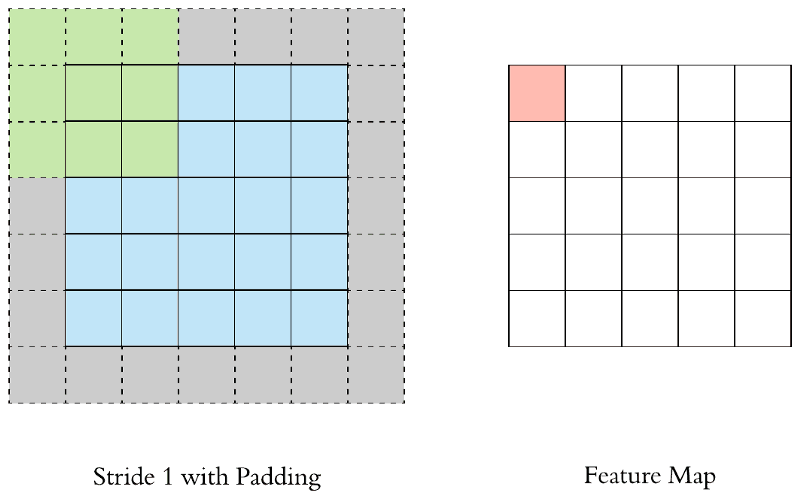This is the core element of the convolution. In practice what happens after the convolution is the creation of as many feature maps as there are filters times channels.

nb feature maps = nb. filter * nb channels

this showed in the following figure:It is also important to point out that the same filters is applied to all the channels. In the above figure, the same 2 filters are applied to the 3 channels resulting in 6 feature maps.

### pooling

Images are high dimensional data structures that need a large amount of computing power to process. One way of reducing this cost is to reduce the reduce the dimensionality of images. One way of reducing dimensionality is through downsampling, which is also called subsampling or max pooling.

The activation maps are fed into a downsampling layer, that split the feature map into patches. Max pooling takes the largest value from each patch of a feature map and places it in a new matrix next to the max values from other patches. This means that the networks will only keep the strongest values and discard the others.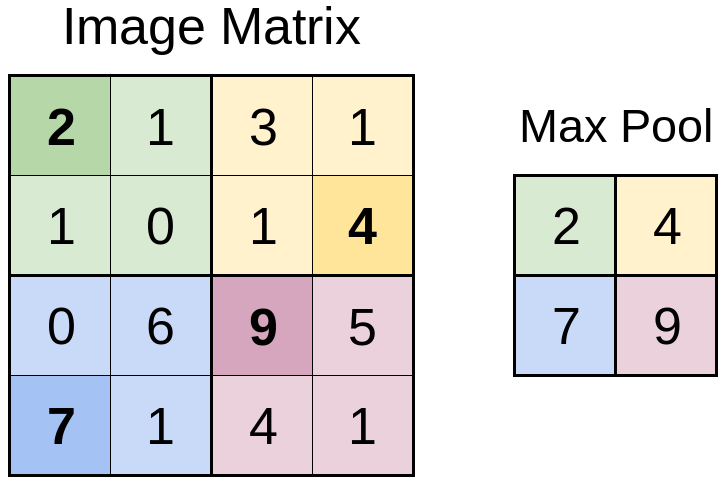The above figure shows the pooling operation on 2×2 patches. Notice how the size is reduced and only max values are kept. Another way of reducing the feature map is through the average pooling operation.

Let’s place the Pooling layers in the context of our Neural Network. This layer come right after the convolution and there will be the same number of max pooled elements as there are feature maps (see figure below)Before continuing to the next layer type, lets note that there typically will be several convolutional layers that can be alternated with max polling layers at any order (see the following figure).### The fully connected layer

The  fully connected layer takes the results of the convolution/pooling process and use them to classify the input into a label.

First, the output of convolution/pooling is flattened into a single vector of values, then these values will feed a fully connected network that will learn to classify this input into a set of categories.

The following figure illustrates how the input values flow into the first layer of neurons and continue into the following layers just like in a classic artificial neural network.How many neurons should be on the fully connected layer? That’s a hyper parameter to tune. All neurons from all the layers in the Convolution/pooling layer are connected to all the neurons on the fully connected layer.

### Softmax/Logistic regression Layer

The final classification occur in this layer. The output layer have one neuron per class. In a classification problem this neuron will fire if the class is predicted. the following figure shows the output layer as a continuation of our neural network. The output neurons will  be either 0 if the class is not predicted or 1 if the class is predicted.

In any classification it is convenient to have not only the exact 0 or 1 prediction, but also  a probability—a value between 0 and 1, exclusive.

The Logistic regression layer take care of producing these probabilities in the case of a 2 classes problem. For example, consider a logistic regression model for a 2 classes problem. If the model infers a value of 0.932 on a particular input, it implies a 93.2% probability that the input is classified in that particular class and 6.8 percent for the competing class. In a logistic regression layer all the probabilities will sum to 1.

Softmax on the other hand,  extends this to a multi-class problem. The probabilities must still add up to 1.0. This additional constraint also helps training converge more quickly.

### Multi-Label Classification

First some mathematical definitions:

Logistic function:

Softmax:

Note that the logistic function is not normalised while the softmax is.

Another constraint that we need to deal with in the output layer, is the multi-label case where each data sample can belong to multiple classes. In this case we can not apply softmax activation, because softmax converts the score into probabilities taking other scores into consideration. There are multiple reasons why we want the final score to be independent as logically items can not logically be constrained into a single class. For example an movie can also belong to the category too. In this case we use the multiple-logistic-regression activation function on the final layer. the sigmoid function (another name for the logistic function) converts each score of the final node between 0 to 1 independent of what the other scores are. Then if the score for a class is more than 0.5, the data is classified into that class. And there could be multiple classes having a score of more than 0.5 independently. The following figure illustrate this difference.CNN was first developed for image classification, but the concepts are generic and can be applied to various domains.

In the next post I will apply them to a text classification problem.